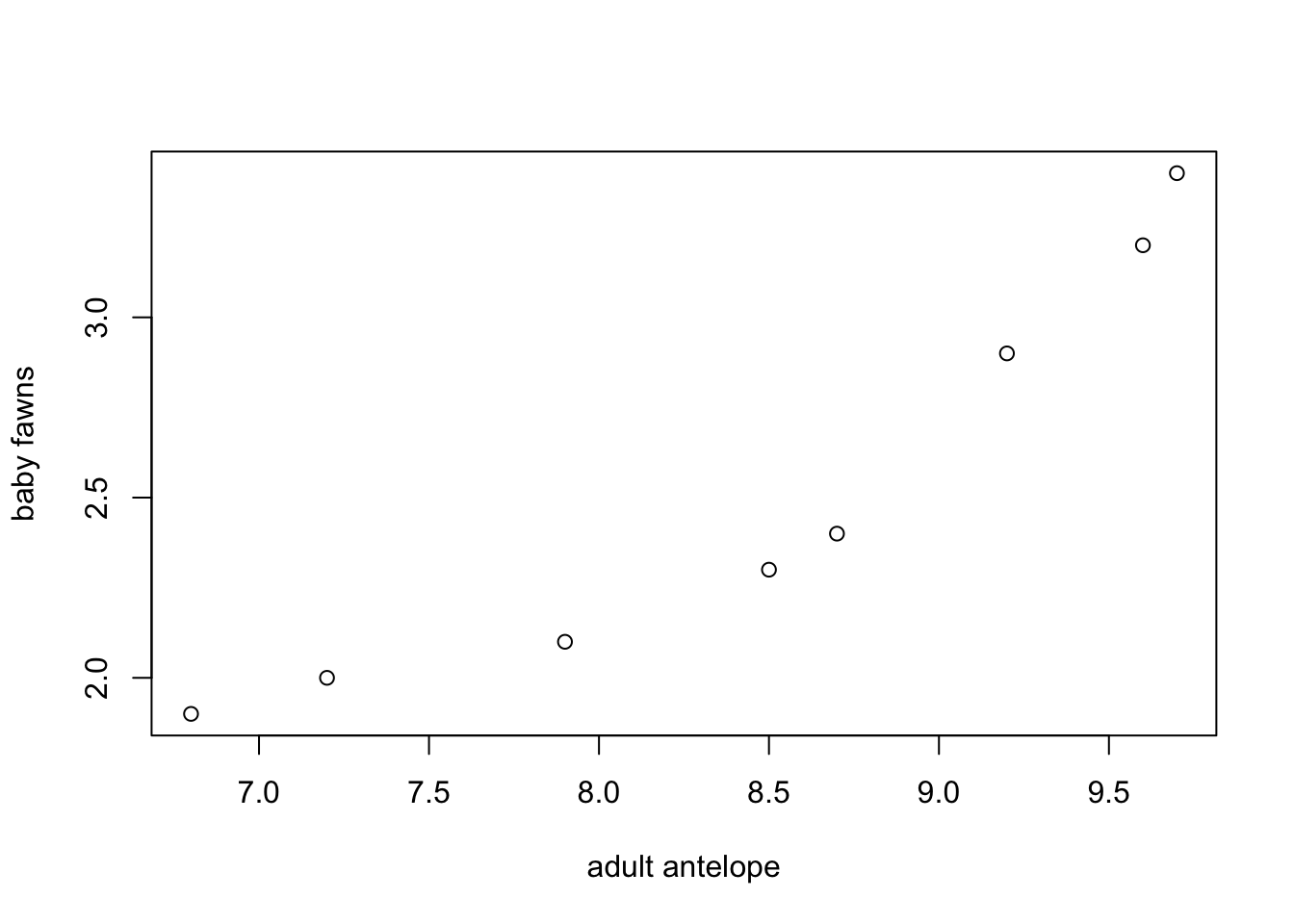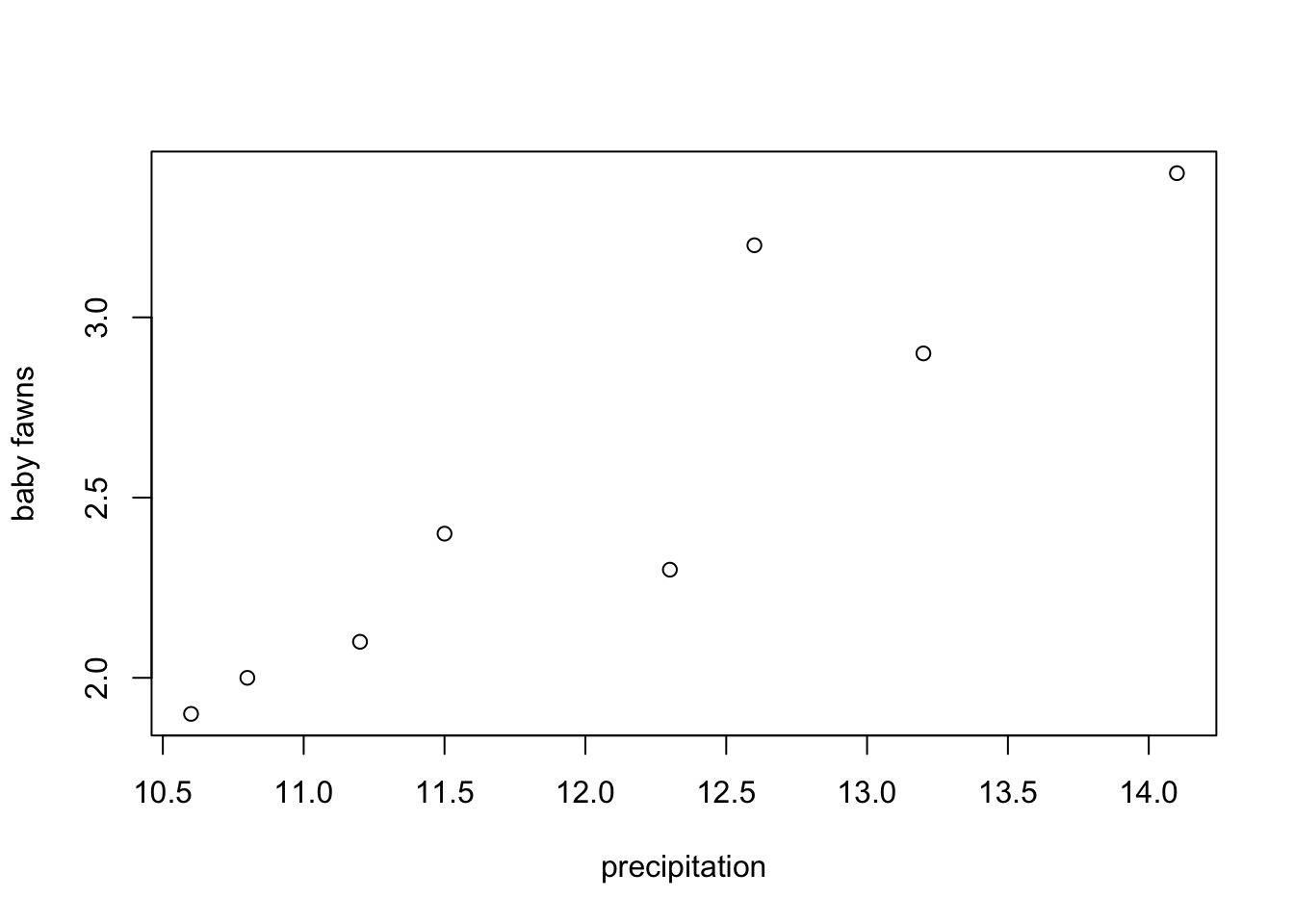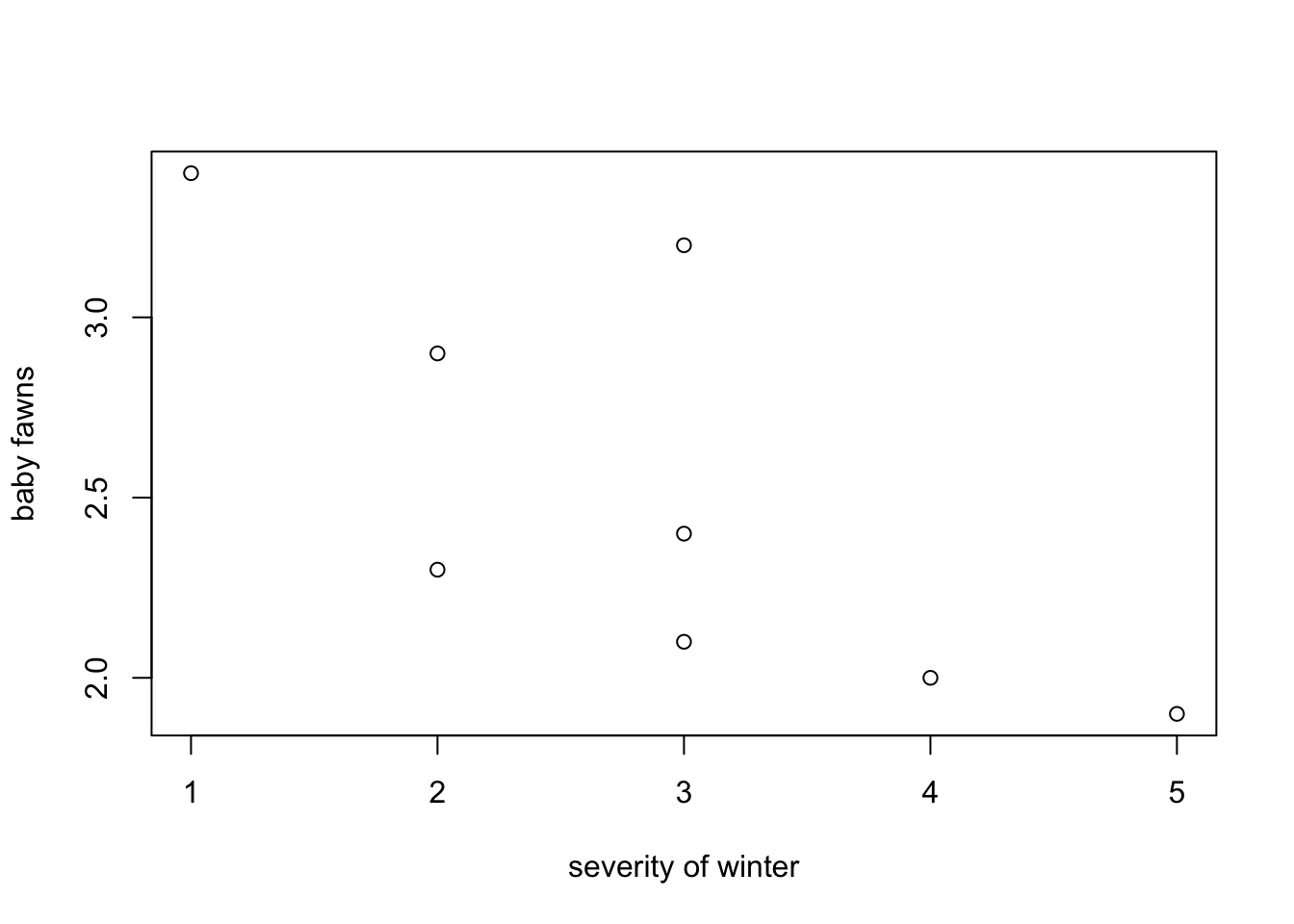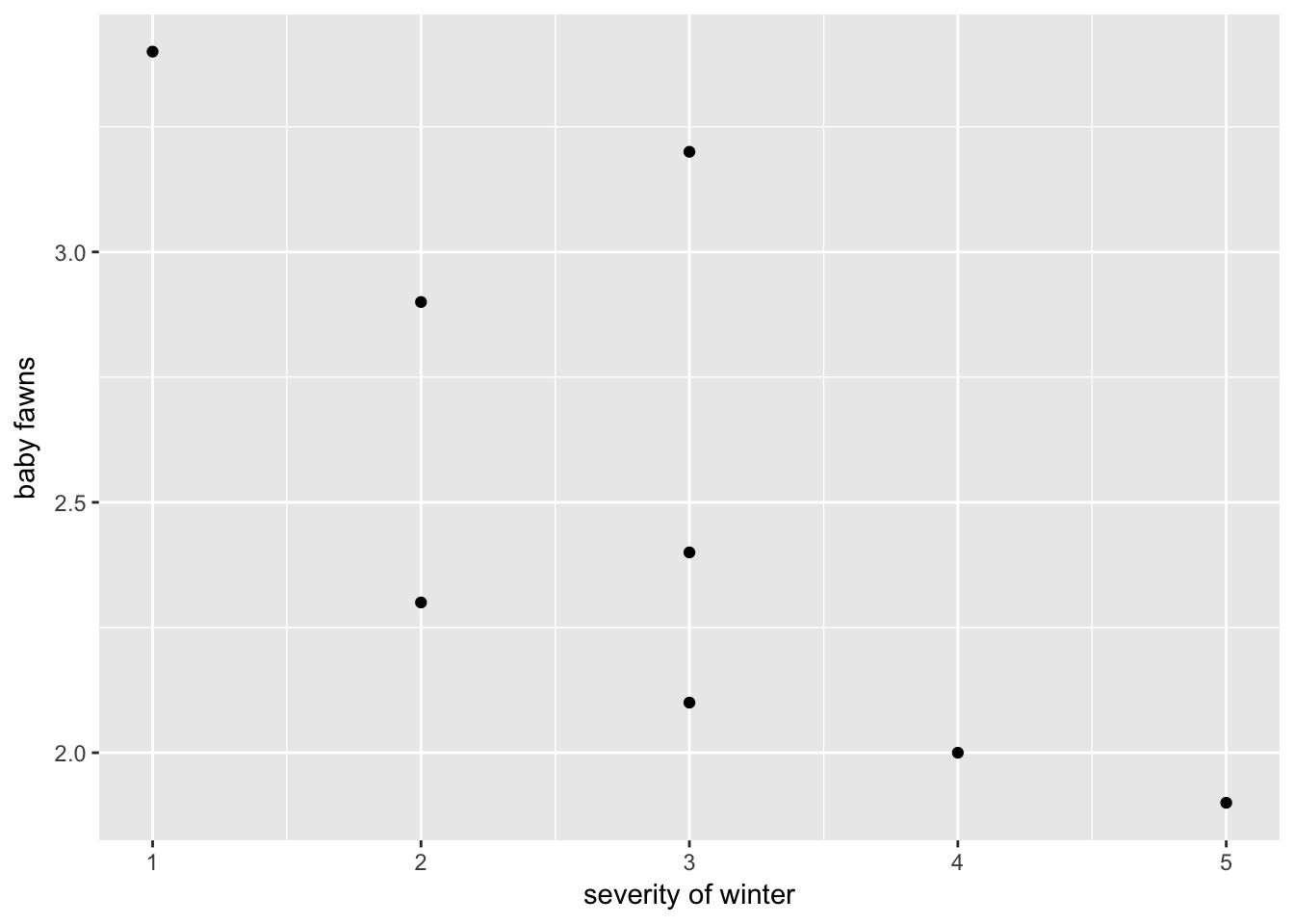# HW 8: Making predictions

## 1. Read in data from URL

`````` # load "gdata" package so that you can read from Excel
# install.packages("gdata")
library(gdata)``````
``## gdata: read.xls support for 'XLS' (Excel 97-2004) files ENABLED.``
``## ``
``## gdata: read.xls support for 'XLSX' (Excel 2007+) files ENABLED.``
``````##
## Attaching package: 'gdata'``````
``````## The following object is masked from 'package:stats':
##
##     nobs``````
``````## The following object is masked from 'package:utils':
##
##     object.size``````
``````## The following object is masked from 'package:base':
##
##     startsWith``````
`````` # read the data directly from the web into our own data frame "df"
# assign column names to the dataset
colnames(df) <- c("fawn", "adult", "precipitation", "severity")``````

## 2. Inspect the data

`````` # make sure that there are 8 rows and 4 columns
str(df)``````
``````## 'data.frame':    8 obs. of  4 variables:
##  \$ fawn         : num  2.9 2.4 2 2.3 3.2 ...
##  \$ adult        : num  9.2 8.7 7.2 8.5 9.6 ...
##  \$ precipitation: num  13.2 11.5 10.8 12.3 12.6 ...
##  \$ severity     : int  2 3 4 2 3 5 1 3``````

## 3. Create bivariate plots of number of baby fawns versus the other three variables

``````library(ggplot2)
# The number of fawns is the outcome (dependent) variable, so it shoulb be Y-axis.
# plot the relationship between fawns and adult antelope`````` # plot the relationship between fawns and precipitation
plot(df\$fawn ~ df\$precipitation, xlab="precipitation", ylab="baby fawns")```````````` # plot the relationship between fawns and the severity of the winter
plot(df\$fawn ~ df\$severity, xlab="severity of winter", ylab="baby fawns")```````` ggplot(df, aes(x=severity, y=fawn)) + geom_point() + xlab("severity of winter") + ylab("baby fawns")```````` ggplot(df, aes(x=severity, y=fawn)) + geom_point(aes(size=adult,color=precipitation)) +
xlab("severity of winter") + ylab("baby fawns")``````